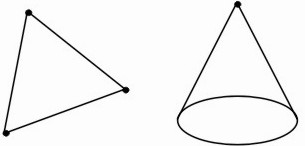HOME MATH DICTIONARY DOWNLOAD FEEDBACK DISCLAIMER
 Question: What is Vertex ? Answer: A vertex of a shape is one of its corners. In a polygon a vertex is the point where two sides meet, while in a polyhedron it is a point where three or more faces or edges meet. The pointed end of a cone is also called its vertex. In graph theory, the points of the graph are called the vertices. We can partially describe a graph by saying how many vertices it has. Example of a Vertex: